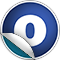# Theory

### Related Docs: trait Theory | package theories

#### object Theory

Linear Supertypes
AnyRef, Any
Ordering
1. Alphabetic
2. By inheritance
Inherited
1. Theory
2. AnyRef
3. Any
1. Hide All
2. Show all
Visibility
1. Public
2. All

### Type Members

1. #### trait Decoder[A] extends AnyRef

In some theories, complex values will internally be encoded as integers.

In some theories, complex values will internally be encoded as integers. Decoders are used to translate back to foreground objects.

3. #### class DefaultDecoderContext extends DecoderContext

Decoder context that will extract all data from the given `model`.

Decoder context that will extract all data from the given `model`.

### Value Members

1. #### final def !=(arg0: Any): Boolean

Definition Classes
AnyRef → Any
2. #### final def ##(): Int

Definition Classes
AnyRef → Any
3. #### final def ==(arg0: Any): Boolean

Definition Classes
AnyRef → Any

5. #### final def asInstanceOf[T0]: T0

Definition Classes
Any
6. #### def clone(): AnyRef

Attributes
protected[java.lang]
Definition Classes
AnyRef
Annotations
@throws( ... )
7. #### final def eq(arg0: AnyRef): Boolean

Definition Classes
AnyRef
8. #### def equals(arg0: Any): Boolean

Definition Classes
AnyRef → Any
9. #### def finalize(): Unit

Attributes
protected[java.lang]
Definition Classes
AnyRef
Annotations
@throws( classOf[java.lang.Throwable] )
10. #### def genAxioms(theoryFunctions: Seq[IFunction] = List(), theoryAxioms: IFormula = IExpression.i(true), extraPredicates: Seq[Predicate] = List(), genTotalityAxioms: Boolean = false, preOrder: TermOrder = TermOrder.EMPTY, functionEnc: FunctionEncoder = new FunctionEncoder(true, false), otherTheories: Seq[Theory] = List()): (Seq[Predicate], Formula, TermOrder, Map[IFunction, Predicate])

Preprocess a set of axioms and convert them to internal representation.

11. #### final def getClass(): Class[_]

Definition Classes
AnyRef → Any
12. #### def hashCode(): Int

Definition Classes
AnyRef → Any
13. #### def iPostprocess(f: IFormula, theories: Seq[Theory], signature: Signature): IFormula

Optionally, a post-processor that is applied to formulas output by the prover, for instance to interpolants or the result of quantifier elimination.

Optionally, a post-processor that is applied to formulas output by the prover, for instance to interpolants or the result of quantifier elimination. This method will be called form within `ap.parser.Postprocessing`.

14. #### def iPreprocess(f: IFormula, theories: Seq[Theory], signature: Signature): (IFormula, Signature)

Apply preprocessing to a formula over some set of theories, prior to sending the formula to a prover.

Apply preprocessing to a formula over some set of theories, prior to sending the formula to a prover. This method will be called form within `ap.parser.Preprocessing`

15. #### final def isInstanceOf[T0]: Boolean

Definition Classes
Any
16. #### def isModelGenPredicate(p: Predicate): Boolean

Test whether `p` belongs to any set `Theory.modelGenPredicates`.

Test whether `p` belongs to any set `Theory.modelGenPredicates`.

17. #### final def ne(arg0: AnyRef): Boolean

Definition Classes
AnyRef
18. #### final def notify(): Unit

Definition Classes
AnyRef
19. #### final def notifyAll(): Unit

Definition Classes
AnyRef
20. #### def postSimplifiers(theories: Seq[Theory]): Seq[(IExpression) ⇒ IExpression]

Compute the list of simplifiers defined by the theories.

21. #### def postprocess(f: Conjunction, theories: Seq[Theory], order: TermOrder): Conjunction

Optionally, a post-processor that is applied to formulas output by the prover, for instance to interpolants or the result of quantifier elimination.

Optionally, a post-processor that is applied to formulas output by the prover, for instance to interpolants or the result of quantifier elimination. This method will be called form within `ap.parser.Postprocessing`.

22. #### def preprocess(f: Conjunction, theories: Seq[Theory], order: TermOrder): Conjunction

Apply preprocessing to a formula over some set of theories, prior to sending the formula to a prover.

23. #### def rewritePreds(f: Conjunction, order: TermOrder)(rewrite: (Atom, Boolean) ⇒ Formula): Conjunction

Apply a uniform substitution to a formula, rewriting atoms to arbitrary new formulas.

Apply a uniform substitution to a formula, rewriting atoms to arbitrary new formulas. TODO: optimise

25. #### final def synchronized[T0](arg0: ⇒ T0): T0

Definition Classes
AnyRef
26. #### def toString(): String

Definition Classes
AnyRef → Any
27. #### final def wait(): Unit

Definition Classes
AnyRef
Annotations
@throws( ... )
28. #### final def wait(arg0: Long, arg1: Int): Unit

Definition Classes
AnyRef
Annotations
@throws( ... )
29. #### final def wait(arg0: Long): Unit

Definition Classes
AnyRef
Annotations
@throws( ... )